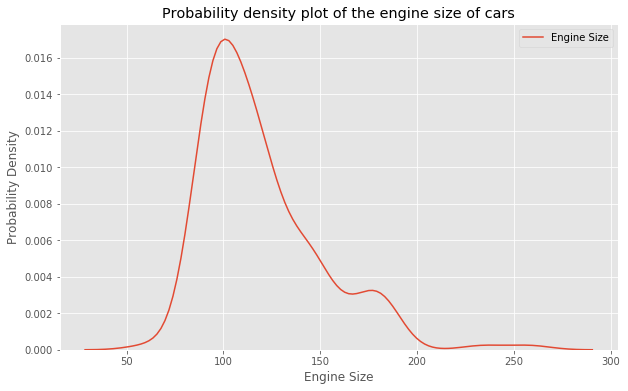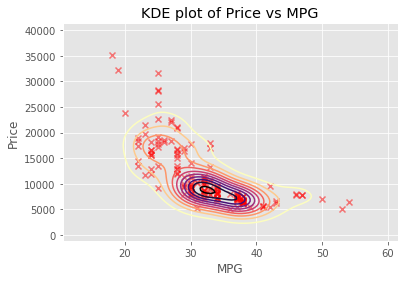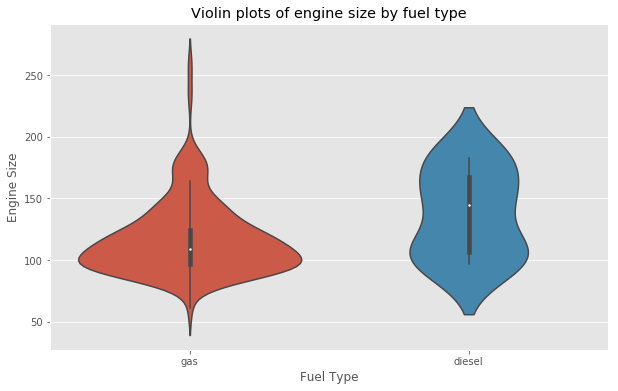# KDE and violin plots using seaborn

In this post we’re going to explore the use of seaborn to make Kernel Density Estimation (KDE) plots and Violin plots.

Both of these plots give an idea of the distribution of your data.

In :
import matplotlib.pyplot as plt
import numpy as np
import pandas as pd
import seaborn as sns

plt.style.use('ggplot')

In :
auto = pd.read_csv('data/auto_prices.csv')
# Drop missing values
auto = auto.replace('?', np.nan).dropna()


## KDE Plots

A KDE plot is a lot like a histogram, it estimates the probability density of a continuous variable.

Let’s take a look at how we would plot one of these using seaborn. We’ll take a look at how engine

In :
plt.figure(figsize=(10,6))
sns.kdeplot(auto['engine-size'], label='Engine Size')
plt.xlabel('Engine Size')
plt.ylabel('Probability Density')
plt.title('Probability density plot of the engine size of cars')
plt.show()## 2D KDE Plots

If we wanted to get a kernel density estimation in 2 dimensions, we can do this with seaborn too.

So if we wanted to get the KDE for MPG vs Price, we can plot this on a 2 dimensional plot.

We’ll also overlay this 2D KDE plot with the scatter plot so we can see outliers.

In :
sns.kdeplot(auto['highway-mpg'], auto['price'], cmap='magma_r')
plt.scatter(auto['highway-mpg'], auto['price'], marker='x', color='r', alpha=0.5)
plt.xlabel('MPG')
plt.ylabel('Price')
plt.title('KDE plot of Price vs MPG')
plt.show()## Violin Plots

A violin plot combines boxplots with KDE plots.

Here we’re going to look at the violin plots of engine size by the fuel type split out into gas and diesel.

In :
plt.figure(figsize=(10,6))
sns.violinplot(x='fuel-type', y='engine-size', data=auto)
plt.title('Violin plots of engine size by fuel type')
plt.xlabel('Fuel Type')
plt.ylabel('Engine Size')
plt.show()